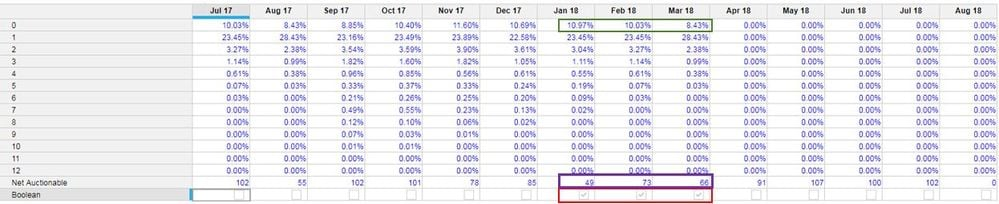# Anchoring Certain Months Data Forward Using BooleanHello All - i am trying to forecast out data using actuals.  I want to take the weighted average of the last three months of actual data (green box below) weighted by net auctionable (purple box below) and forecast out the rest of the year using this average.  I setup a boolean row (red box below) in order to indicate which months I would like to average.  So if we are working on row # 0, the formula would weighted average 10.97%, 10.03% and 8.43% and that number would be populated in the April 18 column and all future months after.  Then we would move to row 1 and do the same thing with 23.45%, 23.45% and 28.43% weighted by net auctionable, and push out that number to April 18 and beyond.

I am having an issue anchoring the weighted averages to only the TRUE columns on the boolean row.  Any help would be very very much appreciated.  Thank youTagged:

•Could you do this by adding some staging line items? I see this being possible with the following steps:

• Create "Staging 0" through "Staging 12" and "Staging Net Auctionable"
• In the "Staging Net Auctinoable" line item, set the formula to "IF Boolean THEN Net Auctionable ELSE 0"
• In the "Staging 0" line item, set the formula to "IF NOT Boolean THEN 0 ELSE '0' * Net Auctionable/Net Auctionable [SELECT: Time.All Periods]"
• Create "Final 0" throgh "Final 12"
• Create a boolean-formatted line item (I'll call it "Future Period" that identifies future periods (or if you have one already somewhere in your model it can just be referenced)
• In the "Final 0" line item, set the formula to "IF Future Period THEN 'Staging 0'[SELECT: Time.All Periods] ELSE '0'"
•If the three months you want to average are always the previous 3 months, then there are simpler ways to do things (instead of using a boolean).  For example, if actuals are loaded through March 18, and you want to forecast April 18 forward, then you can set/hardcode the last month of actuals in one place (or calculate it in one place) rather than setting your booleans... and then calculate the three months.  The weighted average would be a LOOKUP  based formula using the explict 3 month range... rather than trying to ask which months have a TRUE boolean value.

There would therefore be three cells somewhere that each contain a Period value.  One cell would either be manually set by you (i.e. equal to the last period of actuals), or derive based on the switchover (if set) or based on some other way we can tell which is the latest month of actuals.  The remaining 2 cells would be the previous two periods respectively.

Therefore, the averaging formula example might be (I'm too lazy to show your weighted formula, but the idea is the same):  (0[lookup: Month1]+0[lookup: Month2]+0[lookup: Month3])/3... all based off of three cells (somewhere) that tells Anaplan what the target 3 months of actuals are.

If you are using versions w/ a switchover setting, then the attached document contains an example of how you might dynamically identify the 3 months based on the switchover setting (which is version-specific).

Let me know if this helps!

Paul